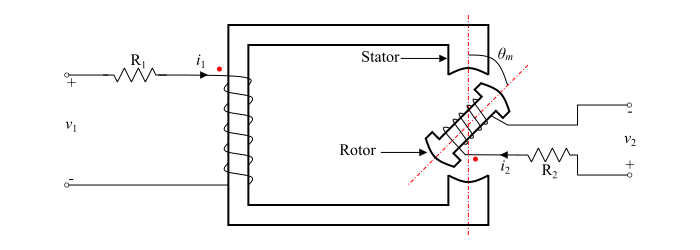# What is a Doubly-Excited System?

Electronics & ElectricalElectronDigital Electronics

#### Ethical Hacking & Cyber Security Online Training

36 Lectures 5 hours

#### Machine Learning & BIG Data Analytics: Microsoft AZURE

46 Lectures 3.5 hours

#### Advance Big Data Analytics using Hive & Sqoop

Best Seller

50 Lectures 4 hours

A doubly-excited system is the type of magnetic system in which two independent coils are used to produce magnetic field. Examples of doubly-excited systems are synchronous machine, separately excited DC machines, loudspeakers, tachometers etc.Consider a doubly-excited system as shown in the figure, it consists of a stator wound with a coil having a resistance of R1 and a rotor wound with a coil of resistance R2. Both the coils are excited by independent voltage sources.

Following assumptions are made to analyse a doubly excited system −

• For any rotor position the relationship between flux-linkage (ψ) and current is linear.

• Hysteresis and eddy current losses are neglected.

• The coils have negligible leakage flux.

• The electric fields are neglected and the magnetic fields are predominating.

The expressions for the flux linkage of the two winding are given by,

$$\mathrm{ψ_{1} = L_{1}i_{1}+ Mi_{2}}$$

$$\mathrm{ψ_{2} = L_{2}i_{2}+ Mi_{1}}$$

By applying KVL, the instantaneous voltage equations for the two coils being,

$$\mathrm{V_{1}=i_{1}R_{1}+\frac{d ψ_{1}}{dt}}$$

$$\mathrm{V_{2}=i_{2}R_{2}+\frac{d ψ_{2}}{dt}}$$

Substituting the values of $ψ_{1}$ and $ψ_{2}$ in the corresponding voltage equations, we get,

$$\mathrm{v_{1}=i_{1}R_{1}+\frac{d}{dt}(L_{1}i_{1}+Mi_{2})=i_{1}R_{1}+\frac{d}{dt}(L_{1}i_{1})+\frac{d}{dt}(Mi_{2})\:\:\:\:\:\:...(1)}$$

$$\mathrm{v_{2}=i_{2}R_{2}+\frac{d}{dt}(L_{2}i_{2}+Mi_{1})=i_{1}R_{1}+\frac{d}{dt}(L_{1}i_{1})+\frac{d}{dt}(Mi_{1})\:\:\:\:\:\:...(2)}$$

Since, the inductances are dependent on the position of the rotor angle $θ_{m}$ which is a function of time and are independent of the currents. Similarly, the currents are the function of time and are independent of inductances. Therefore, the eq. (1) and (2) can be written as,

$$\mathrm{v_{1}=i_{1}R_{1}+L_{1}\frac{d i_{1}}{dt}+i_{1}\frac{dL_{1}}{dt}+M\frac{d i_{2}}{dt}+i_{2}\frac{d M}{dt}\:\:\:\:\:\:...(3)}$$

$$\mathrm{v_{2}=i_{2}R_{2}+L_{2}\frac{d i_{2}}{dt}+i_{2}\frac{dL_{2}}{dt}+M\frac{d i_{2}}{dt}+i_{1}\frac{d M}{dt}\:\:\:\:\:\:...(4)}$$

Now, multiplying eqn. (3) by $i_{1}$ and eqn. (4) by $i_{2}$, we get

$$\mathrm{v_{1}i_{1}=i_{1}^{2}R_{1}+L_{1}i_{1}\frac{d i_{1}}{dt}+i_{1}^{2}\frac{dL_{1}}{dt}+Mi_{1}\frac{d i_{2}}{dt}+i_{1}i_{2}\frac{d M}{dt}\:\:\:\:\:\:...(5)}$$

$$\mathrm{v_{2}i_{2}=i_{2}^{2}R_{1}+L_{2}i_{2}\frac{d i_{2}}{dt}+i_{2}^{2}\frac{dL_{1}}{dt}+Mi_{2}\frac{d i_{2}}{dt}+i_{1}i_{2}\frac{d M}{dt}\:\:\:\:\:\:...(6)}$$

The eqns.(5) and (6) are the power expressions for the two coils.

Now, integrating eqns.(5) and (6) with respect to time and adding, we have,

$$\mathrm{\int(v_{1}i_{1}+v_{2}i_{2})dt=\int(i_{1}^2R_{1}+i_{1}^2R_{1})dt+\int(l_{1}i_{1}di_{1}+l_{2}i_{2}di_{2}+i_{1}^2dL_{1}+i_{2}^2dL_{2}+Mi_{1}di_{2}+Mi_{2}di_{1}+2i_{1}i_{2}dM)\:\:\:\:\:\:...(7)}$$

The eqn.(7) is the energy equation for the doubly-excited magnetic system. It shows that the total electrical energy input to the system is equal to the sum of two parts, where the first part is the energy to electrical losses and the second is useful electrical energy, i.e.

$$\mathrm{[Total \:Electrical \:Energy\: Input\: W_{e}] = [Energy\: to\: Electrical\: Losses \:W_{electric.losses}]+[Useful \:Electrical \:Energy\: W_{f}+W_{m}]}$$

Where,

$$\mathrm{W_{f}+W_{m}=\int(l_{1}i_{1}di_{1}+l_{2}i_{2}di_{2}+i_{1}^2dL_{1}+i_{2}^2dL_{2}+Mi_{1}di_{2}+Mi_{2}di_{1}+2i_{1}i_{2}dM)\:\:\:\:\:\:...(8)}$$

## Energy Stored in the Magnetic Field

The instantaneous value of energy stored in the magnetic field depends upon the inductance and values of current at that instant. For any stationary position of the rotor, the mechanical output is zero, and all the useful electrical energy input is converted into field energy. As the inductance values are constant. Therefore, terms $dL_{1}$, $dL_{2}$ and dM become zero. Hence, from eqns.(8), we get,

$$\mathrm{\int dW_{f}=\int_{0}^{i_{1}}L_{1}i_{1}di_{1}+\int_{0}^{i_{2}}L_{2}i_{2}di_{2}+\int_{0}^{i_{1},i_{2}}(Mi_{2}di_{1}+Mi_{1}di_{2})}$$

$$\mathrm{w_{f}=\frac{1}{2}L_{1}i_{1}^2+\frac{1}{2}L_{2}i_{2}^2+Mi_{1}i_{2}\:\:\:\:\:\:...(9)}$$

## Electromagnetic Torque

When the rotor rotates, the rate of change of field energy with respect to time is given by differentiating the eqn.(9),

$$\mathrm{\frac{d w_{f}}{dt}=\frac{1}{2}L_{1}\frac{d i_{1}^{2}}{dt}+\frac{1}{2}i_{1}^2\frac{dL_{1}}{dt}+\frac{1}{2}L_{2}\frac{d i_{2}^{2}}{dt}+\frac{1}{2}i_{2}^2\frac{dL_{2}}{dt}+i_{1}i_{2}\frac{dM}{dt}+Mi_{1}\frac{di_{2}}{dt}+Mi_{2}\frac{di_{1}}{dt}}$$

$$\mathrm{\Longrightarrow\frac{d w_{f}}{dt}=L_{1}i_{1}\frac{di_{1}}{dt}\frac{1}{2}i_{1}^2\frac{dL_{1}}{dt}+L_{2}i_{2}\frac{di_{2}}{dt}+\frac{1}{2}i_{1}^2\frac{dL_{1}}{dt}+\frac{1}{2}L_{2}\frac{di_{2}^{2}}{dt}+\frac{1}{2}i_{2}^2\frac{dL_{2}}{dt}+i_{1}i_{2}\frac{dM}{dt}+Mi_{1}\frac{di_{2}}{dt}+Mi_{2}\frac{di_{1}}{dt}\:\:\:\:\:\:...(10)}$$

Integrating the eqn.(10) with respect to time, we get,

$$\mathrm{W_{f}=\int L_{1}i_{1}d_{1}+\frac{1}{2}i_{1}^2dL_{1} \int L_{2}i_{2}d_{2}+\frac{1}{2}i_{2}^2dL_{2}+i_{1}i_{2}dM+Mi_{1}di_{2}+Mi_{2}di_{1}\:\:\:\:\:\:...(11)}$$

The eqn. (11) is a general equation for a moving transducer in which $L_{1}$, $L_{2}$, $M, i_{1}$ and $i_{2}$ are all varying with position and time. Now, comparing eqn.(11) with eqn.(8), we obtain,

$$\mathrm{W_{m}=\int(\frac{1}{2}i_{1}^2dL_{1}+\frac{1}{2}i_{2}^2dL_{2}+i_{1}i_{2}dM+)\:\:\:\:\:\:...(12)}$$

Differentiating eqn.(12) with respect to rotor angle $θ_{m}$, we get,

$$\mathrm{\frac{dW_{m}}{dθ_{m}}=\int(\frac{1}{2}i_{1}^2\frac{dL_{1}}{dθ_{m}}+\frac{1}{2}i_{2}^2\frac{dL_{2}}{dθ_{m}}+i_{1}i_{2}\frac{dM}{dθ_{m}})}$$

$$\mathrm{\Longrightarrow \tau_{e}=\frac{1}{2}i_{1}^2\frac{dL_{1}}{dθ_{m}}+\frac{1}{2}i_{2}^2\frac{dL_{2}}{dθ_{m}}+i_{1}i_{2}\frac{dM}{dθ_{m}}\:\:\:\:\:\:...(13)}$$

The first two terms of the equation (13) are the reluctance torque and the last term is known as the co-alignment torque, that is due to the two superimposed magnetic fields try to align.

Important − For the machines having uniform air gaps the reluctance torque is not produced.

## Numerical Example

For a doubly excited system, the inductances are given as follows −

$$\mathrm{L_{1}=10+2cos2θ;L_{2}=5+2cos2θ;M=20cosθ}$$

The coils are excited by current $i_{1}$=0.5A and $i_{2}$=0.6A.

• Find the torque as a function of θ.

• Find the energy stored in the system as a function of θ.

## Solution

• The torque produced in a doubly-excited system is given by

$$\mathrm{\tau_{e}=\frac{1}{2}i_{1}^2\frac{dL_{1}}{dθ_{m}}+\frac{1}{2}i_{2}^2\frac{dL_{2}}{dθ_{m}}+i_{1}i_{2}\frac{dM}{dθ_{m}}}$$

here,

$$\mathrm{\frac{dL_{1}}{dθ_{m}}=-4sin2θ;\frac{dL_{2}}{dθ_{m}}=-6sin2θ;=-20sinθ}$$

$$\mathrm{\Longrightarrow\tau_{e}=\frac{1}{2}\times(0.5)^2\times(-4sin2θ)+\frac{1}{2}\times(0.6)^2\times(-6sin2θ)+(0.5)\times(0.6)\times-20sinθ}$$

$$\mathrm{\therefore\tau_=-1.58sin2θ-6sinθNm}$$

• The energy stored in doubly-excited system is given by,

$$\mathrm{W_{f}=\frac{1}{2}L_{1}i_{1}^2+\frac{1}{2}L_{2}i_{2}^2+Mi_{1}i_{2}}$$

$$\mathrm{\Longrightarrow W_{f}=\frac{1}{2}(10+2cos2θ)(0.5)^2+\frac{1}{2}(5+2cos3θ)(0.6)^2+(20cosθ)(0.5)(0.6)}$$

$$\mathrm{\Longrightarrow W_{f}=2.15+0.79cos2θ+6cosθ}$$